Function Repository Resource:

# CurveFitOverview

Get an association of information related to fitting a set of data

Contributed by: Wolfram|Alpha Math Team
 ResourceFunction["CurveFitOverview"][data,var,type] performs a numeric fit of the specified type to data with respect to the user-specified variable x, and returns an Association of the result.

## Details and Options

type can be "Linear", "Quadratic", "Cubic", "Quartic", "Quintic", "Logit", "Probit", "Exponential", "Log", "Power" or {"Polynomial",power} for a given integer power.

## Examples

### Basic Examples (3)

Compute information about a power fit to a given set of data:

 In:=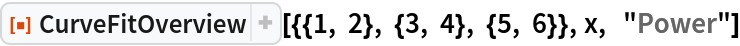Out=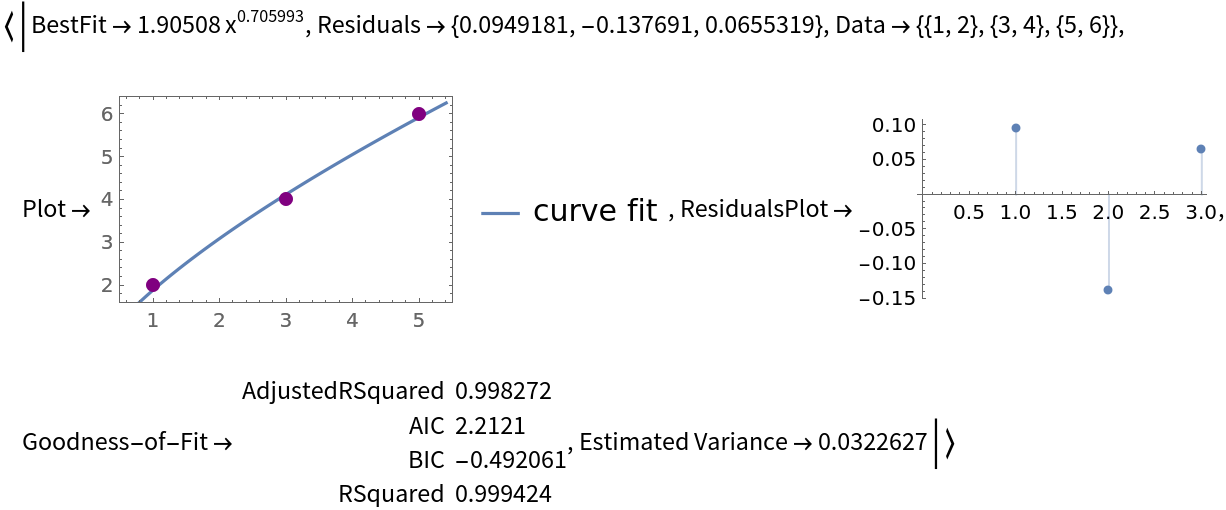Compute information about a second degree polynomial fit to a given set of data:

 In:=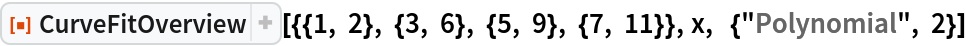Out=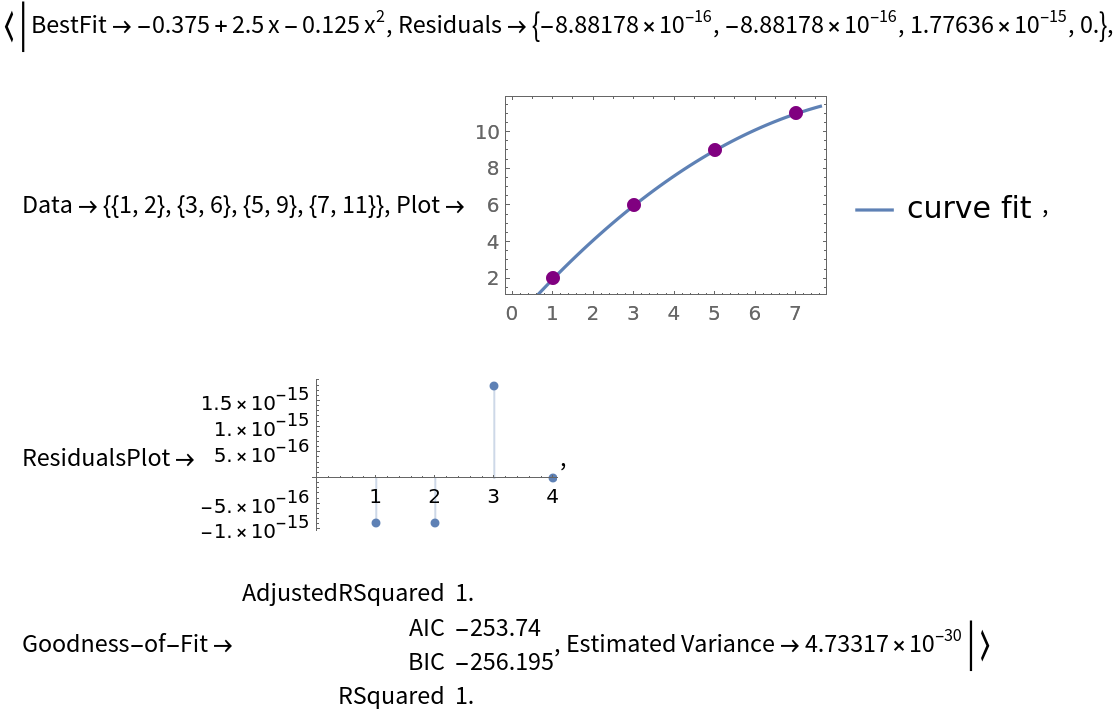Compute information about an exponential fit to a given set of data:

 In:=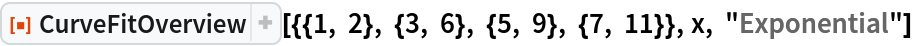Out=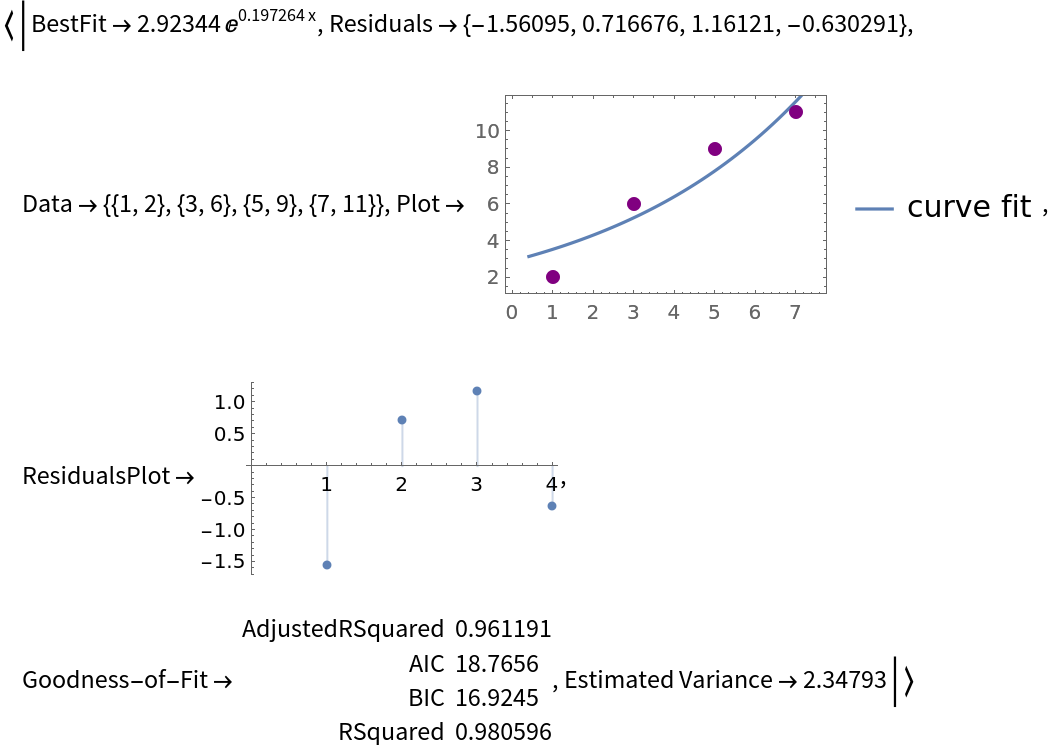### Properties and Relations (1)

The information returned by CurveFitOverview can be visualized as a report using the resource function CurveFitReport:

 In:=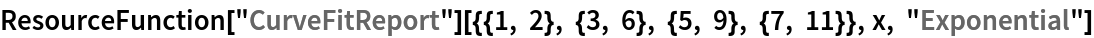Out=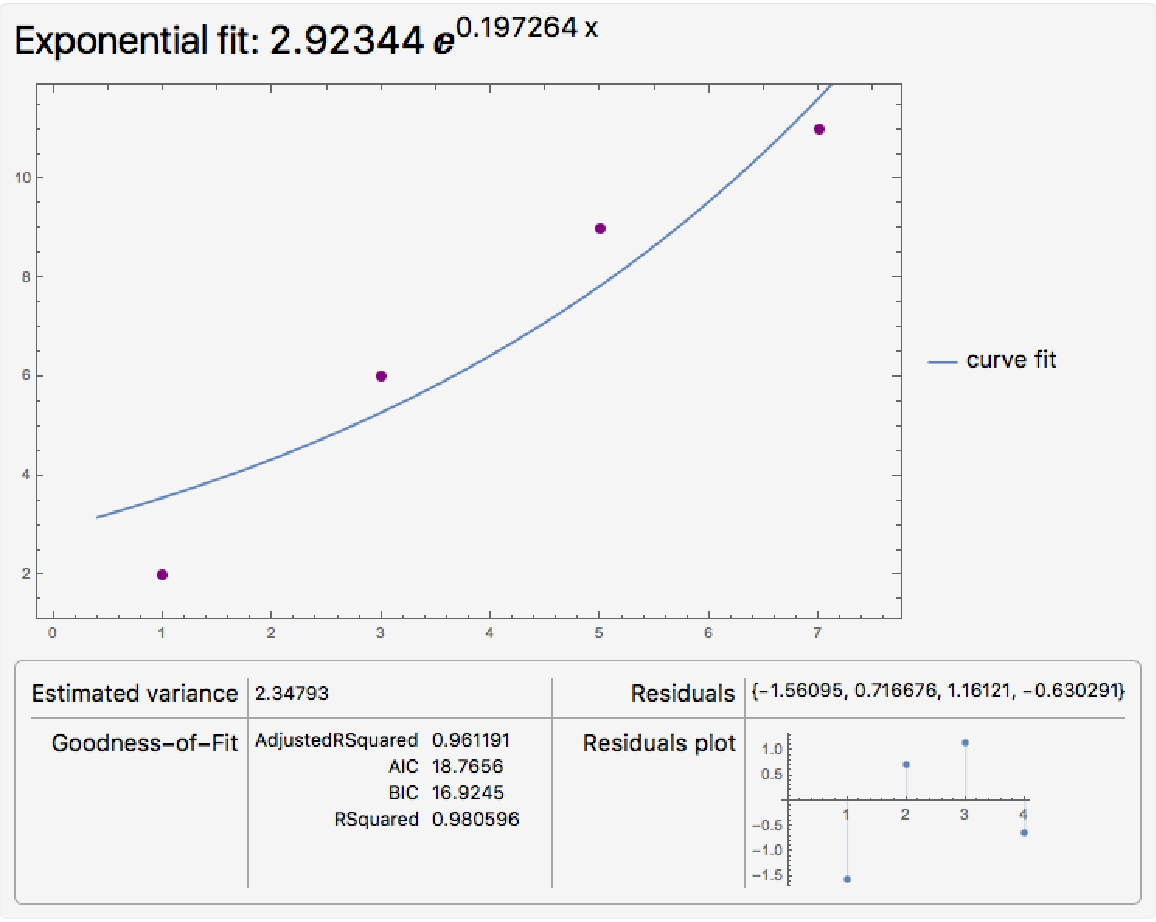### Possible Issues (1)

CurveFitOverview will return unevaluated if the specified data is not compatible with the given type:

 In:=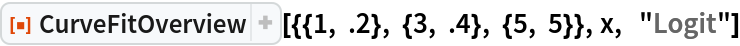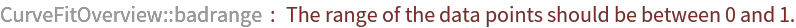Out=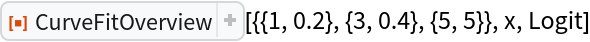## Publisher

Wolfram|Alpha Math Team

## Version History

• 2.0.0 – 23 March 2023
• 1.0.0 – 10 April 2020